## Practice Questions – Analogy- 1

1. If  each consonant in the word TOLERANT is replaced by the previous letter in the English alphabet and each vowel in the word is replaced by the next letter in the English alphabet and the new set of letters are arranged alphabetically, which of the following will be the fourth from the right after the replacement?

(a) M

(b) P

(c) Q

(d) K

(e) None of these

2. How many such As are there in the above arrangement each of which is immediately preceded by B and also immediately followed by a consonant?

(a) None

(b) One

(c) Two

(d) Three

(e) More than three

3. If all the vowels (in all the vowels) are changed to the next letter of the English alphabetical series, how many words will form meaningful English words?

(a) One

(b) Two

(c) Three

(d) More than three

(e) None

4. How many meaningful English words can be formed-not ending with E-with the second, the fourth the sixth and the ninth letter of the word PROACTIVE? (To be counted from left side)

(a) One

(b) Two

(c) Three

(d) Four

(e) More than four

5. How many meaningful English words can be formed, starting with S, with the second, the fourth, the fifth and the eighth letters of the PERISHED, using each letter only once in each word? (To be counted from left)

(a) None

(b) One

(c) Two

(d) Three

(e) More than three

6. How many such pairs of letters are there in the word STREAMING each of which has as many letters between them in the word as in the English alphabet? (in both forward and backward directions)

(a) None

(b) One

(c) Two

(d) Three

(e) More than three

7. If it is possible to make only one meaningful English word with the second, the third, the seventh and the eighth letters of the word STEADFAST, which of the following will be the second letter of that word? If no such word can be formed, give ‘X’ as the answer and if more than one such word can be formed, give ‘Y’ as the answer

(a) E

(b) A

(c) T

(d) X

(e) Y

8. How many such pairs of letters are there in the word BARGAINED, each of which has as many letters between them in the word (in both forward and backward directions) as they have between them in the English alphabetical series?

(a) Two

(b) Three

(c) None

(d) One

(e) More than three

9. If each alternate letter in the word ‘FLIPPER’ starting with F is changed to the next letter in the English alphabetical series and each of the remaining letters is changed to the previous letters in the English alphabetical series, then how many letters will appear more than once in the new arrangement?

(a) None

(b) One

(c) Two

(d) Three

(e) Four

10. How many meaningful English words can be formed with the letters CFAE using each letter only once in each word?

(a) None

(b) One

(c) Two

(d) Three

(e) More than three

Explanation

1. (b)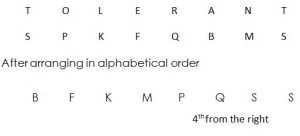2. (d)

 B A Consonant

C E B A C D B C D A C E D E

D C A B A D A C E D U B A N B D

3. (e)

PIN=PJN;

ROD=DOR

DEN=DFN

SAT=SBT

FUN=FVN

4. (a)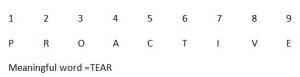5. (b)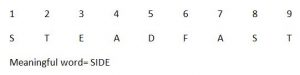6. (d)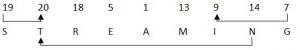7. (e)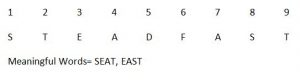8. (e)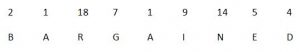9. (a)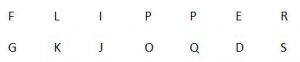10. (c)

Meaningful words = FACE, CAFE